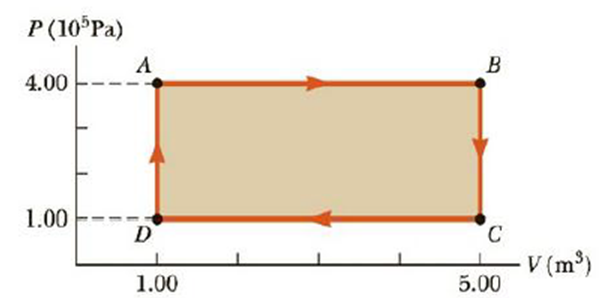Chapter 12, Problem 6P

Chapter
Section
Textbook Problem

A gas follows the PV diagram in Figure P12.6. Find the work done on the gas along the paths (a) AB, (b) BC, (c) CD, (d) DA, and (e) ABCDA.Figure P12.6

(a)

To determine
The work done along the path AB.

Explanation

Given Info:

Constant pressure of the gas is 4×105Pa

Initial volume ( Vi ) of the gas is 1m3 .

Finial volume ( Vf ) of the gas is 5m3 .

Formula to calculate the work done of the gas is,

W=P(VfVi)

• P is the pressure
• VfVi is the change in volume

Substitute 4

(b)

To determine
The work done along the path BC.

(c)

To determine
The work done along the path CD.

(d)

To determine
The work done along the path DA.

(e)

To determine
The work done along the path ABCDA.

Still sussing out bartleby?

Check out a sample textbook solution.

See a sample solution

The Solution to Your Study Problems

Bartleby provides explanations to thousands of textbook problems written by our experts, many with advanced degrees!

Get Started